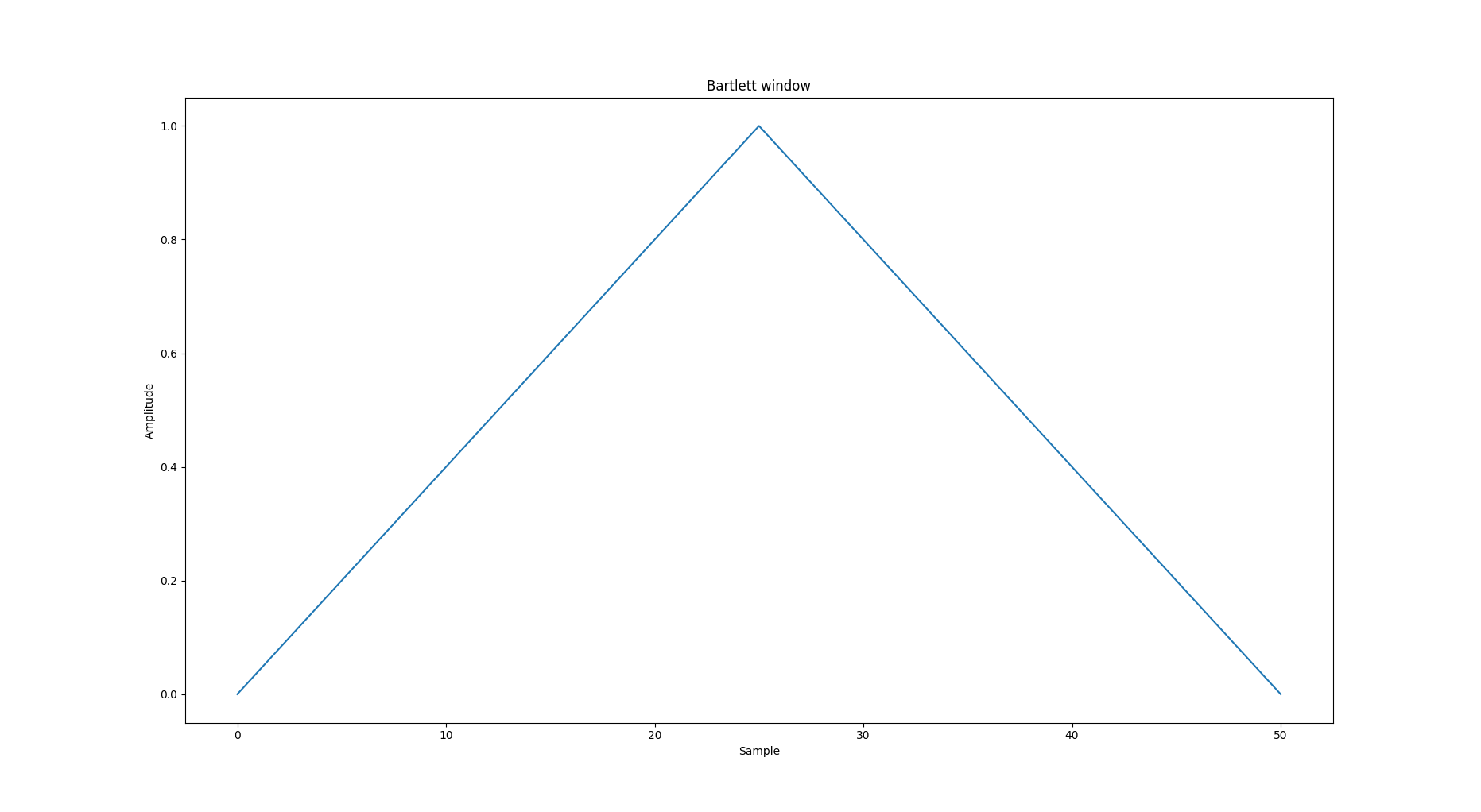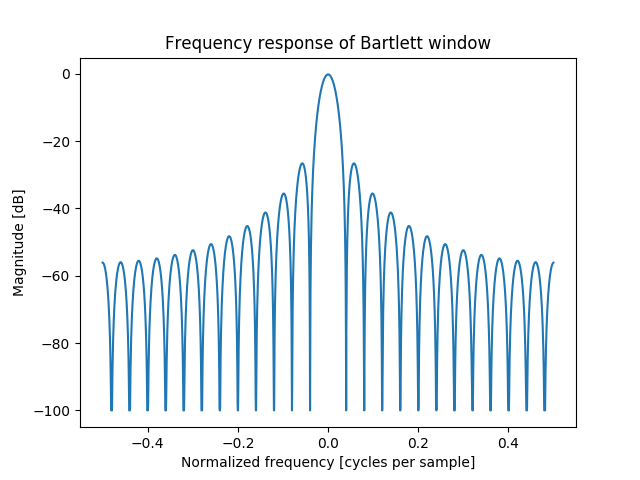# numpy bartlett() in Python

The Bartlett window is very similar to a triangular window, except that the endpoints are at zero. It is often used in signal processing for tapering a signal, without generating too much ripple in the frequency domain.

```Parameters(numpy.bartlett(M)):
M : int Number of points in the output window.
If zero or less, an empty array is returned.

Returns:
out : array```

The triangular window, with the maximum value normalized to one (the value one appears only if the number of samples is odd), with the first and last samples equal to zero.

Example:

 `import` `numpy as np ` `print``(np.bartlett(``12``)) `

Output:

```[ 0.          0.18181818  0.36363636  0.54545455  0.72727273  0.90909091
0.90909091  0.72727273  0.54545455  0.36363636  0.18181818  0.        ]

```

Plotting the window and its frequency response (requires SciPy and matplotlib):
For Window:

 `import` `numpy as np ` `import` `matplotlib.pyplot as plt ` `from` `numpy.fft ``import` `fft, fftshift ` `window ``=` `np.bartlett(``51``) ` `plt.plot(window) ` `plt.title(``"Bartlett window"``) ` `plt.ylabel(``"Amplitude"``) ` `plt.xlabel(``"Sample"``) ` `plt.show() `

Output:For frequency:

 `import` `numpy as np ` `import` `matplotlib.pyplot as plt ` `from` `numpy.fft ``import` `fft, fftshift ` `window ``=` `np.bartlett(``51``) ` `plt.figure() ` `A ``=` `fft(window, ``2048``) ``/` `25.5` `mag ``=` `np.``abs``(fftshift(A)) ` `freq ``=` `np.linspace(``-``0.5``, ``0.5``, ``len``(A)) ` `response ``=` `20` `*` `np.log10(mag) ` `response ``=` `np.clip(response, ``-``100``, ``100``) ` `plt.plot(freq, response) ` `plt.title(``"Frequency response of Bartlett window"``) ` `plt.ylabel(``"Magnitude [dB]"``) ` `plt.xlabel(``"Normalized frequency [cycles per sample]"``) ` `plt.axis(``'tight'``) ` `plt.show() `

Output:My Personal Notes arrow_drop_upCheck out this Author's contributed articles.

If you like GeeksforGeeks and would like to contribute, you can also write an article using contribute.geeksforgeeks.org or mail your article to contribute@geeksforgeeks.org. See your article appearing on the GeeksforGeeks main page and help other Geeks.

Please Improve this article if you find anything incorrect by clicking on the "Improve Article" button below.

Article Tags :

Be the First to upvote.

Please write to us at contribute@geeksforgeeks.org to report any issue with the above content.